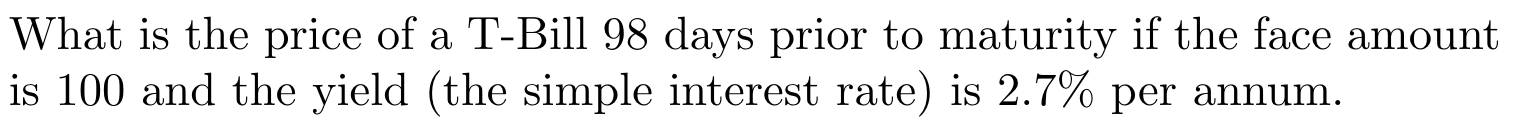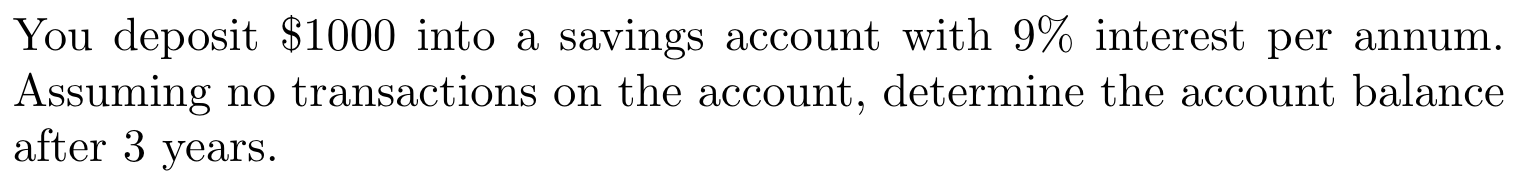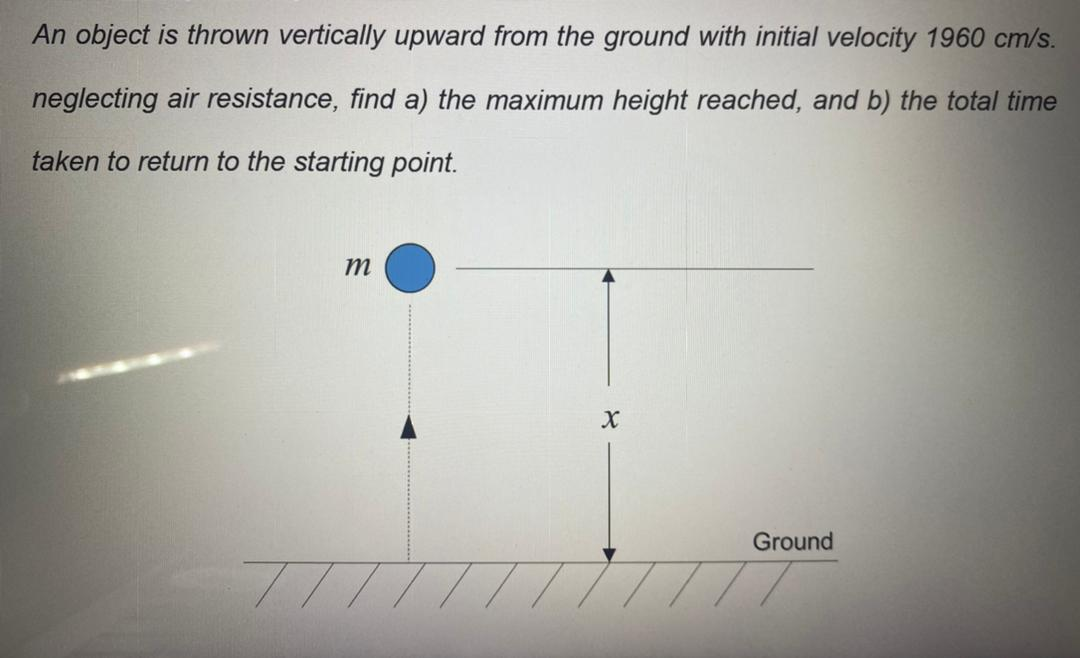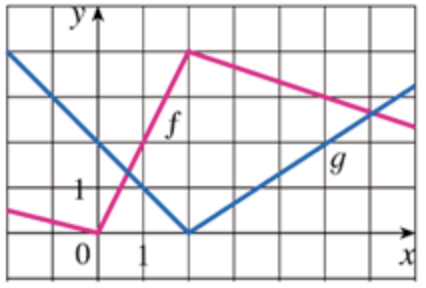##### Function and tengent line

For what value of h will the line y=-2x+h be tangent to y=3x2+4x-1?

b) Graph the line and the curve on the same set of axes to verify the solution. Use a scale.

In: Math

##### Price of T-Bill

What is the price of a T-Bill 98 days prior to maturity if the face amount is 100 and the yield (the simple interest rate) is 2.7% per annum.In: Math

##### Account Balance after 3 years

You deposit \$1000 into a saving account with 9% interest per annum. Assuming no transactions on the account, determine the account balance after 3 years.In: Math

##### Ordinary Differential equation (Newton's Law)

An object is thrown vertically upward from the ground with an initial velocity of 1960 cm/s. neglecting air resistance, find a) the maximum height reached, and b) the total time is taken to return to the starting point.In: Math

##### Percentage and Applications

A sum of Rs.6000 is borrowed at 10% pa compounded interest and paid back in three equal annual instalments.

What is the approximate amount of each instalment?

In: Math

##### General solution of PDE

Find the general solution of the PDE.

$$y^{\prime \prime}-6 y^{\prime}+9 y=0$$

In: Math

##### Real analysis

The Couchy product of two absolutely convergent series converges absolutely.

In: Math

##### Y varies directly as the square of X. If Y = 144 when X = 6, what is the value of y when x = 3

Y varies directly as the square of x. If y = 144 when x = 6, what is the value of y
when x = 3

In: Math

Find f'(x)

In: Math

##### What is the Derivative of f(x)=(4x^7)^5

Find the Derivative of the f(x)

In: Math

##### If f(x) = exg(x), where g(0) = 3 and g'(0) = 4, find f '(0).

If $$f(x)=e^{x} g(x),$$ where $$g(0)=3$$ and $$g(0)=4,$$ find $$f(0)$$

In: Math

##### Find equations of the tangent lines to the curve y = (x-1)/(x+1) that are parallel to the line x − 2y = 5.

Find equations of the tangent lines to the curve y = (x-1)/(x+1) that are parallel to the line x − 2y = 5.

In: Math

##### Let P(x) = F(x)G(x) and Q(x) = F(x)/G(x), where F and G are the functions whose graphs are shown.

Let P(x) = F(x)G(x) and Q(x) = F(x)/G(x), where F and G are the functions whose graphs are shown.
(a) Find P ' (2)
(b) Find Q ' (7)

In: Math

##### if f and g are the functions whose graphs are shown, let u(x) = f(x)g(x) and v(x) = f(x)/g(x).

if f and g are the functions whose graphs are shown, let u(x) = f(x)g(x) and v(x) = f(x)/g(x)(a) Find u'(1)

(b) Find v'(5).

In: Math